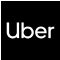New update is available. Click here to update.
Topics

# Roots of the tree having minimum height

Moderate0/80
Average time to solve is 25m+2 more companies

## Problem statement

You are given an undirected Tree having 'N' nodes. The nodes are numbered from 1 to 'N'. Your task is to find the sorted list of all such nodes, such that rooting the tree on that node gives the minimum height possible of the given tree.

A tree is a connected acyclic graph. The height of a rooted tree is the maximum value of the distance between the root and all nodes of the tree.

Detailed explanation ( Input/output format, Notes, Images )
Constraints:
``````1 <= T <= 10
1 <= N <= 10^5
1 <= X,Y <= N

The input edges form a tree.

Time limit: 1 sec
``````
Sample Input 1:
``````2
3
1 2
2 3
4
1 2
3 1
4 1
``````
Sample Output 1:
``````2
1
``````
Explanation for Sample Input 1:
``````For the first test case, if we root the tree at Node 2, the height of the resultant tree is 1 which is the minimum possible height.
For the second test case, if we root the tree at Node 1, the height of the resultant tree is 1 which is the minimum possible height.
``````
Sample Input 2:
``````2
4
1 3
2 3
4 2
2
1 2
``````
Sample Output 2:
``````2 3
1 2
``````Console